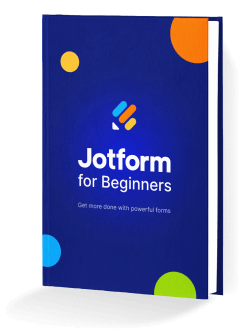Last Update:

Logical functions in Advanced Formula are used to test whether a logical expression or argument is true or false. Depending on the result of that test, you can then elect to do one thing or another and conditionally display information, perform different calculations, or perform further tests.

Notes: In Jotform Tables, TRUE (bool) outputs either 1 or “true”, while FALSE (bool) outputs either 0 or an empty string.

Here’s the list of logical functions you can use in Jotform Tables:

Function Description
AND Returns TRUE if all the arguments are true, returns FALSE otherwise.
BLANK Returns a blank value.
ERROR Returns the error value.
FALSE Returns the logical value FALSE.
IF Returns the first statement if the logical argument is true, returns the second statement otherwise.
ISERROR Returns TRUE if the expression causes an error.
NOT Reverses the logical value of its argument.
OR Returns TRUE if any of the arguments is true.
SWITCH Similar to a series of IF conditions on the same expression.
TRUE Returns the logical value TRUE.
XOR Returns a logical exclusive OR of all arguments.

## AND

Returns FALSE if at least one of its logical arguments is false.

Syntax:

``AND(logical1,logical2[,logical3,...])``

Examples:

• `=AND(TRUE(),TRUE())` will return TRUE — all arguments are true.
• `=AND(TRUE(),FALSE())` will return FALSE — one of the arguments is false.
• `=AND(FALSE(),FALSE())` will return FALSE — all arguments are false.
• `=AND({Number}>=1,{Number}<=10)` will return TRUE if the value of `Number` is between 1 and 10.
• `=AND("Hello","World")` will return FALSE — invalid arguments.

## BLANK

Returns an empty value. The BLANK function has no arguments.

Syntax:

``BLANK()``

Examples:

• `=BLANK()` will return an empty string.
• `=""=BLANK()` will return TRUE — “” is an empty string.
• `=" "=BLANK()` will return FALSE — white space is not considered empty.
• `=0=BLANK()` will return FALSE — zero is not considered empty.
• `={Answer}=BLANK()` will return TRUE if `Answer` is empty.

## ERROR

Returns the “#ERROR!” value. The ERROR function has no arguments.

Syntax:

``ERROR()``

Examples:

• `=ERROR()` will return #ERROR!.
• `=IF({Answer}=BLANK(),ERROR(),"OK!")` will return #ERROR! if `Answer` is empty.

## FALSE

Returns the logical value FALSE. The FALSE function has no arguments.

Syntax:

``FALSE()``

Examples:

• `=FALSE()` will return FALSE.
• `=AND({Answer}="Yes",FALSE())` will return FALSE.
• `=NOT(FALSE())` will return TRUE.
• `=IF({Number}=0,FALSE(),TRUE())` will return FALSE if the value of `Number` is 0 and TRUE otherwise.

## IF

Returns or executes the first statement if the logical argument is true; returns or executes the second statement if the logical argument is false.

Syntax:

``IF(logical,statement1,statement2)``

Examples:

• `=IF(TRUE(),"Yes","No")` will return Yes.
• `=IF(FALSE(),"Yes","No")` will return No.
• `=IF(100=100,"Equal","Not Equal")` will return Equal.
• `=IF(100>0,2+2,3+3)` will return 4.
• `=IF({Age}>=18,"Approved","Denied")` will return Approved if the value of `Age` is greater than or equal to 18 and Denied otherwise.

## ISERROR

Returns TRUE if the expression causes an error.

Syntax:

``ISERROR(expression)``

Examples:

• `=ISERROR(ERROR())` will return TRUE — #ERROR! error.
• `=ISERROR({Number}/0)` will return TRUE — #DIV/0! error.
• `=ISERROR("Sunday"+1)` will return TRUE — #NUM! error.
• `=ISERROR(FIND("needle","in this haystack"))` will return TRUE — #VALUE! error.
• `=IF(ISERROR("Power"=9000),"Error","Valid")` will return Valid — although “Power” is not equal to 9000, `"Power"=9000` is a valid (no error) logical expression.

## NOT

Returns TRUE if the logical argument is false; returns FALSE if the logical argument is true.

Syntax:

``NOT(logical)``

Examples:

• `=NOT(TRUE())` will return FALSE.
• `=NOT(FALSE())` will return TRUE.
• `=NOT(100=100)` will return FALSE.
• `=NOT("Apples"="Oranges")` will return TRUE.
• `=NOT("Hello World")` will return #VALUE! — invalid argument.

## OR

Returns TRUE at least one of its logical arguments is true.

Syntax:

``OR(logical1,logical2[,logical3,...])``

Examples:

• `=OR(TRUE(),TRUE())` will return TRUE — all arguments are true.
• `=OR(TRUE(),FALSE())` will return TRUE — one of the arguments is true.
• `=AND(FALSE(),FALSE())` will return FALSE — all arguments are false.
• `=OR({Number}<1,{Number}>10)` will return FALSE if the value of `Number` is between 1 and 10.
• `=OR("Hello","World")` will return FALSE — invalid arguments.

## SWITCH

Evaluates a value or expression against a list of possible values, and it returns the result corresponding to the first matching value. If there is no match, an optional default value may be returned.

Syntax:

``SWITCH(expression,value1,result1[,default|value2,result2{,...}])``

Examples:

• `=SWITCH(2+3,5,"Correct","Incorrect")` will return Correct.
• `=SWITCH({Answer},"Yes","Approved","No","Denied","Undecided")` will return Approved if the value of `Answer` is “Yes”, Denied if “No,” and Undecided if neither.
• `=SWITCH({Number},1,"One",2,"Two",3,"Three","No Match")` will return One, Two, or Three if the value of `Number` is 1, 2, or 3 respectively. It will return No Match if no match was found.

## TRUE

Returns the logical value TRUE. The TRUE function has no arguments.

Syntax:

``TRUE()``

Examples:

• `=TRUE()` will return TRUE.
• `=AND({Answer}="Yes",TRUE())` will return TRUE if the value of `Answer` is “Yes.”
• `=NOT(TRUE())` will return FALSE.
• `=IF({Number}=0,TRUE(),FALSE())` will return TRUE if the value of `Number` is 0.

## XOR

Returns TRUE if an odd number of arguments are true, regardless of the number of false arguments.

Syntax:

``XOR(logical1[,logical2,...])``

Examples:

• `=XOR(TRUE(),FALSE(),FALSE())` will return TRUE — one (odd) argument is true.
• `=XOR(TRUE(),TRUE())` will return FALSE — two (even) arguments are true.
• `=XOR(TRUE(),TRUE(),TRUE())` will return TRUE — three (odd) arguments are true.
• `=XOR(TRUE(),TRUE(),FALSE())` will return FALSE — two (even) arguments are true.
• `=XOR(FALSE(),FALSE())` will return FALSE — all arguments are false.
Contact Support:

Our customer support team is available 24/7 and our average response time is between one to two hours.
Our team can be contacted via:

Contact Jotform Support: https://www.jotform.com/contact/

## Send Comment: